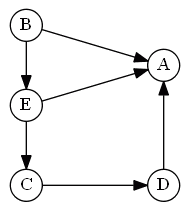# ML Wiki

## ELECTRE

This is a method from the family of outranking (i.e. based on pair-wise comparison) MCDA methods. The result is a partial ordering of alternatives (see Partial Order Preference Structure)

Here:

• ELECTRE I

ELECTRE I chooses alternatives that are preferred by the majority of the criteria and which don;t cause an unacceptable level of discontentment on other criteria.

## Preferences

Main idea
When we say $a \ P \ b$?
• (1) if we have a lot of criteria for which $u_i(a) > u_i(b)$ (where $u_i$ - some utility function)
• (2) and we have no strong arguments that say the opposite of (1)

The ELECTRE methods are based on two concepts:

• Concordance Index
• Discordance Index

### Concordance Index

Or quantification of positive arguments

For ELECTRE 1, when comparing $a$ and $b$

• identify all criteria $g_j$ such that $g_j(a) \geqslant g_j(b)$
• for all such criteria $g_j$ sum their weights $w_j$,
• let $W$ be the sum of all weights: $W = \sum_i w_i$ (we use this for normalization)
• define the concordance index as:
$c(a, b) = \cfrac{1}{W} \sum_{j: g_j(a) \geqslant g_j(b)} w_j$

Note that weight should sum up to 1

There are two extreme cases:

• $c(a, b) = 1$: all criteria for $a$ are better than for $b$ (Unanimity)
• have very good reasons to say $a \ P \ b$
• $c(a, b) = 0$: there is no criteria in which $a$ better than $b$

### Discordance Index

Or quantification of negative arguments

We want to find a strong argument against $a \ P \ b$

• if we find such an argument then we cannot say that $a \ P \ b$

For ELECTRE I, define $d(a, b)$ as

• 0 when $\forall j: g_j(a) \geqslant g_j(v)$ (none when there's Unanimity)
• if $\exists g_i: g_i(b) \geqslant g_i(a)$ then we have an argument against $a \ P \ b$
• in this case we want to identify the largest difference between $a$ and $b$:
• $\cfrac{1}{\delta} \max_j [g_j(b) - g_j(a)]$
• $\delta$ is normalization factor: $\delta = \max_{i,c,d} [g_i(c) - g_i(d)]$

Another way

• let $D$ be the set of cases where we list cases that cannot be compared
• if for a pair of alternatives $(a, b)$ there exists a criteria $g_i$ s.t. $(g_i(a), g_i(b)) \in D$ then we cannot compare these alternatives

### Outranking Preference Relation

For ELECTRE I we define $S \equiv P \lor I$ as: (the "as good as relations", also see Voting Theory Relations)

• $a \ S \ b \iff$
• (1) $c(a, b) \geqslant \tilde c$
a lot of positive arguments to say $a \ S \ b$
• (2) $d(a, b) \leqslant \tilde d$
not many negative arguments to say the opposite
• so for that we also have to provide two parameters:
• $\tilde c$ - the concordance threshold and $\tilde d$ - the discordance threshold

Or:

• $a \ S \ b \iff$
• (1) $c(a, b) \geqslant \tilde c$
• (2) $\forall g_i: \big(g_i(a), g_i(b)\big) \not \in D$

Note that this relation gives us partial order:

• we have $P$ and $I$ (expressed via $S$)
• and we have $J$ (when discordance threshold is exceeded)

## Graph Kernels

Graph Kernels are used to identify good alternatives.

It is possible to express the outranking relation $S$ in form of a directed graph

Example:

•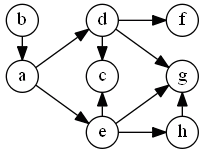• $a$ is better than $e$ and $d$
• $d$ is better than $f$ and $c$
• etc

A kernel of a graph $K \subset A$ is

• $\forall a \in K, \not \exists b: a \ S \ b$
no alternative $a$ inside the kernel $K$ is better than any other alternative $b$ inside $K$
• $\forall a,b \in K: a \ ? \ b$
within the kernel we cannot say anything about the relation between $a$ and $b$
• $\forall c \not \in K, \exists a \in K: a \ S \ c$
each alternative $c$ outside of the kernel $K$ is worse than at least one alternative $a$ inside $K$

For the example above:

• $K = \{b, d, e\}$
•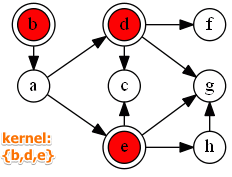Another example

•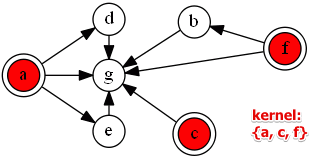Remarks:

The kernel $K$ of a set $A$ forms a set of preferred alternatives.

## Examples

### Example 1

price comfort speed aesthetic
1 300 ex fast good
2 250 ex med good
3 250 med fast good
4 200 med fast med
5 200 med med good
7 100 poor med med
$w$ 5 4 3 3 $W = \sum w = 15$

#### Concordance

Perform pair-wise comparisons for all elements $A$

• let's compare (1) and (2)
• concordance: $c(1, 2) = \cfrac{1}{15} (4 + 3 + 3) = \cfrac{2}{3}$

This way we compare each with each:

1 2 3 4 5 6 7
1 - 10 10 10 10 10 10
2 12 - 12 7 10 7 10
3 11 11 - 10 10 10 10
4 8 8 12 - 12 12 10
5 8 11 12 12 - 12 10
6 11 11 11 11 11 - 10
7 5 8 5 8 8 9 -

(note that this is not normalized - need also to divide on 15)

#### Discordance

In this case we define discordance by enumerating cases that are forbidden

The following tables shows what veto we define

price comfort
$a$ 250 poor
$b$ 100 excellent

#### Graph

So the establish the following relation $S$ and the following graph: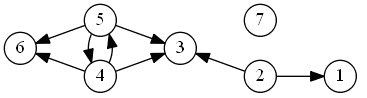There are two kernels in this case:

• 2, 4, 7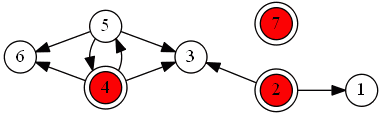• 2, 5, 7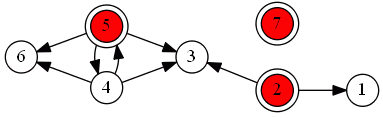Kernel:

• 6 is dominated - remove it
• same for 3 and 1
• since there's a cycle we have to decide between 4 and 5, and remove one of them
• when we put alternatives into a kernel, it doesn't mean they are the best, but it means it allows to apply the Dominance principle and remove dominated alternatives

### Example 2

• A company wants to rank 5 candidates $A,B,C,D,E$ for a promotion
• Criteria:
• Reputation of diploma $D$
• Skills $K$
• Personality $P$
• Spoken Languages $L$
• Seniority $S$
• Apply ELECTRE with concordance threshold 0.6 and discordance 6

The following evaluation table is obtained

$A$ $B$ $C$ $D$ $E$ Weight
$D$ 7 11 15 11 16 15
$K$ 12 18 6 8 10 15
$P$ 13 13 14 19 10 15
$L$ 18 16 19 13 19 25
$S$ 10 20 16 14 20 20

Total weight is $W = 15 + 15 + 15 + 25 + 20 = 100$

#### Concordance

Then we construct the concordance index by comparing alternatives pair-wise

For example, consider alternatives $A$ and $B$

$A$ vs $B$ $B$ vs $A$
$D$ 15
$K$ 15
$P$ 25 25
$L$ 25
$S$ 20
Total 50 100
Normalized 0.5 0.75

Repeating this for all pairs, construct the following table:

$A$ $B$ $C$ $D$ $E$
$A$ - 0.75 0.15 0.4 0.4
$B$ 0.5 - 0.35 0.75 0.6
$C$ 0.85 0.65 - 0.6 0.5
$D$ 0.6 0.25 0.4 - 0.25
$E$ 0.6 0.6 0.75 0.75 -

Bold are alternatives that should be preferred provided that there's no discordance

• recall that $\tilde c = 0.6$ and we check all values that $\geqslant \tilde c$

#### Discordance

The discordance index is $\tilde d = 6$

• we don't normalize on $\delta$ here because our values are already normalized

Let's compare $A$ with $B$ and $A$ with $C$:

$A$ vs $B$ $B$ vs $A$ $A$ vs $C$ $C$ vs $A$
$D$ 7 - 8 -
$K$ 6 - - 6
$P$ 0 0 1 -
$L$ - 2 1 -
$S$ 10 - 6 -
Max 10 2 8 6
Rel $A \ J \ B$ $A \ J \ C$ $C \ J \ A$
• if a difference exceeds the threshold, the alternatives are incomparable

#### Graph

Based on the Concordance and Discordance we define the outranking relation $S$

• this relation can be depicted as a graph
•